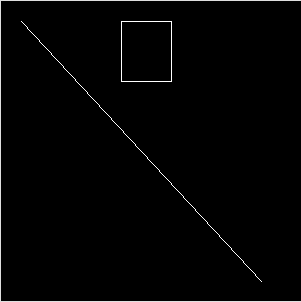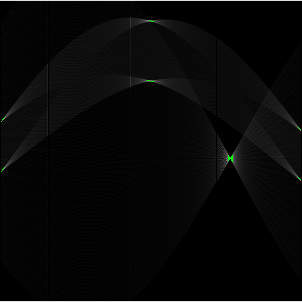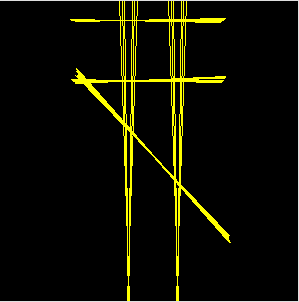## line detection with hough-transform

I wrote a small processing sketch to work out how linedetection using hough-transform works.

click here to see it in action and get the sourcecode. the code is not optimised for speed but for my understanding :-) so don't complain if it doesn't work on live-video.

the algorithm takes a image that has run through an edge detection algorithm and turned into a black/white image and find where things like edges or circles are by converting the pixels from imagespace to a parameterspace where such detection is much easyer.

for each pixel that is set in the source image a set of parameters that satisfy the formular r = x * cos(roh) + y * sin(roh) is calculated and r and roh are plotted as the parameterspace image

every line in the input image results in a bunch of lines in the parameterspace that have a common intersection point.

in my example i use a input image like thiscalculate how it looks in parameter spaceand then reconstruct where the lines are